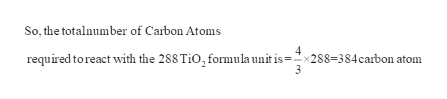# Based on the balanced equation3TiO2 + 4C + 6Cl2 → 2CO2 + 2CO + 3TiCl4calculate the number of of excess reagent units remaining when 288 TiO2 formula units and 388 C atoms react?

Question
36 views

Based on the balanced equation

3TiO2 + 4C + 6Cl2 → 2CO2 + 2CO + 3TiCl4

calculate the number of of excess reagent units remaining when 288 TiO2 formula units and 388 C atoms react?

check_circle

Step 1

The given Balanced chemical Equation is,

Step 2

Calculation For the total number Carbon atom required:

From the balanced chemical equ...help_outlineImage TranscriptioncloseSo, the totalnumber of Carbon Atoms 4 x288=384carbon atom 3 required to react with the 288 TiO, formula unit is fullscreen

### Want to see the full answer?

See Solution

#### Want to see this answer and more?

Solutions are written by subject experts who are available 24/7. Questions are typically answered within 1 hour.*

See Solution
*Response times may vary by subject and question.
Tagged in

### Other